# The MODEL Procedure

### Testing for Normality

The NORMAL option in the FIT statement performs multivariate and univariate tests of normality.

The three multivariate tests provided are Mardia’s skewness test and kurtosis test (Mardia, 1970) and the Henze-Zirkler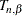test (Henze and Zirkler, 1990). The two univariate tests provided are the Shapiro-Wilk W test and the Kolmogorov-Smirnov test. (For details on the univariate tests, refer to "Goodness-of-Fit Tests" section in "The UNIVARIATE Procedure" chapter in the Base SAS Procedures Guide.) The null hypothesis for all these tests is that the residuals are normally distributed.

For a random sample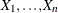,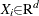, where d is the dimension of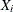and n is the number of observations, a measure of multivariate skewness is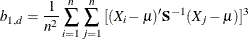where S is the sample covariance matrix of X. For weighted regression, both S and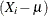are computed by using the weights supplied by the WEIGHT statement or the _WEIGHT_ variable.

Mardia showed that under the null hypothesis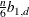is asymptotically distributed as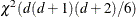. For small samples, Mardia’s skewness test statistic is calculated with a small sample correction formula, given by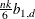where the correction factor k is given by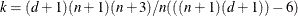. Mardia’s skewness test statistic in PROC MODEL uses this small sample corrected formula.

A measure of multivariate kurtosis is given by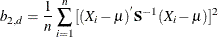Mardia showed that under the null hypothesis,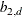is asymptotically normally distributed with mean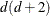and variance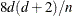.

The Henze-Zirkler test is based on a nonnegative functional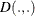that measures the distance between two distribution functions and has the property that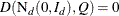if and only if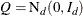where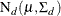is a d-dimensional normal distribution.

The distance measurecan be written as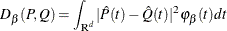where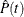and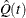are the Fourier transforms of P and Q, and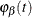is a weight or a kernel function. The density of the normal distribution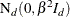is used as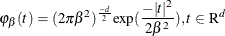where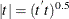.

The parameter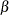depends onas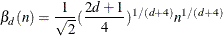The test statistic computed is called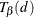and is approximately distributed as a lognormal. The lognormal distribution is used to compute the null hypothesis probability.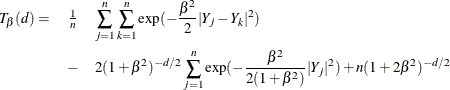where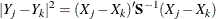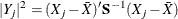Monte Carlo simulations suggest thathas good power against distributions with heavy tails.

The Shapiro-Wilk W test is computed only when the number of observations (n ) is less than 2000 while computation of the Kolmogorov-Smirnov test statistic requires at least 2000 observations.

The following is an example of the output produced by the NORMAL option.


proc model data=test2;
y1 = a1 * x2 * x2 - exp( d1*x1);
y2 = a2 * x1 * x1 + b2 * exp( d2*x2);
fit y1 y2 / normal ;
run;


Figure 19.40: Normality Test Output

The MODEL Procedure

Normality Test
Equation Test Statistic Value Prob
y1 Shapiro-Wilk W 0.34 <.0001
y2 Shapiro-Wilk W 0.82 <.0001
System Mardia Skewness 286.4 <.0001
Mardia Kurtosis 31.28 <.0001
Henze-Zirkler T 6.65 <.0001